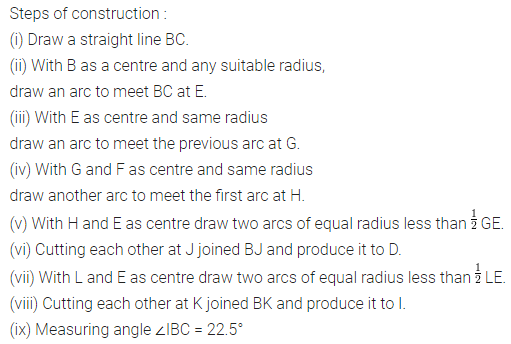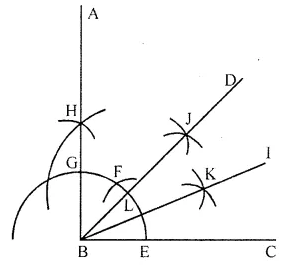ML Aggarwal Class 6 Solutions Chapter 13 Practical Geometry Ex 13.3 for ICSE Understanding Mathematics acts as the best resource during your learning and helps you score well in your exams.

## ML Aggarwal Class 6 Solutions for ICSE Maths Chapter 13 Practical Geometry Ex 13.3

Question 1.
Draw an angle of 80° and make a copy of it using ruler and compass.
Solution: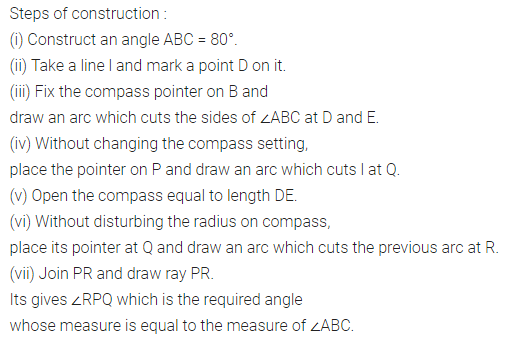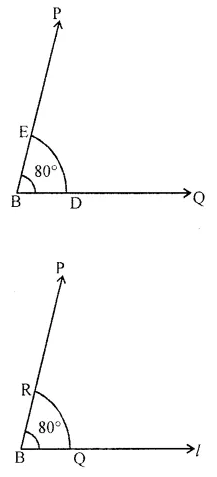Question 2.
Draw an angle of measure 127° and construct its bisector.
Solution: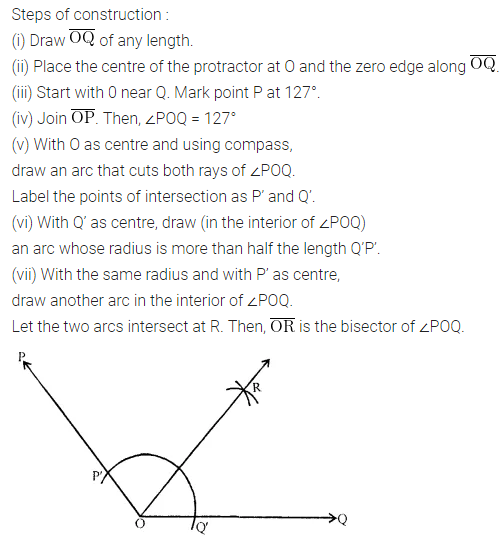Question 3.
Draw ∠POQ = 64°. Also, draw its line of symmetry.
Solution:Question 4.
Draw a right angle and construct its bisector.
Solution: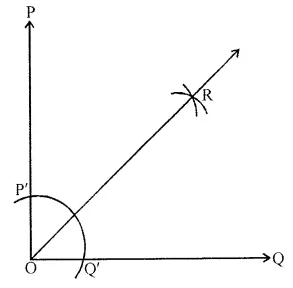Question 5.
Draw an angle of 152° and divide it into four equal parts.
Solution: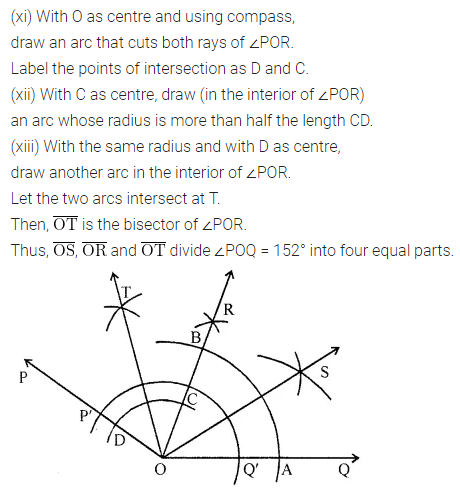Question 6.
Draw an angle of measure 45° and bisect it.
Solution: